﻿ FSD and its Modifications for Shell Finite Elements

### FSD and its Modifications for Shell Finite Elements

Milan Sága, Peter Pecháč, Milan VaškoOPEN ACCESSPEER-REVIEWED

## FSD and its Modifications for Shell Finite Elements

Milan Sága1,, Peter Pecháč1, Milan Vaško1

1Department of Applied Mechanics, Faculty of Mechanical Engineering, Univerzity of Žilina, Žilina, Slovak Republic

### Abstract

Our paper deals with theoretical and numerical study of the discrete fully stress design (FSD) algorithms for thin shell finite elements. Authors present seven mathematical formulations of the direct strength designing. Relations between stresses, membrane and bending element forces (moments) and element thicknesses are studied. Using selected numerical tests of the proposed algorithms are compared.

### At a glance: Figures

123456
Prev Next

• Sága, Milan, Peter Pecháč, and Milan Vaško. "FSD and its Modifications for Shell Finite Elements." American Journal of Mechanical Engineering 1.7 (2013): 427-433.
• Sága, M. , Pecháč, P. , & Vaško, M. (2013). FSD and its Modifications for Shell Finite Elements. American Journal of Mechanical Engineering, 1(7), 427-433.
• Sága, Milan, Peter Pecháč, and Milan Vaško. "FSD and its Modifications for Shell Finite Elements." American Journal of Mechanical Engineering 1, no. 7 (2013): 427-433.

 Import into BibTeX Import into EndNote Import into RefMan Import into RefWorks

### 1. Introduction

The contemporary problems solution of technological practice is closely connected with computer technology. The most sophisticated engineering tasks are nowadays solved thanks to the development of algorithmized numerical procedures and computers efficiency. Nowadays a new branch of science “Simulation-Based Engineering Science”, which simulates engineering systems behaviour on the basis of mathematical methods and computers, is going into the foreground.

Here the simulation is not understood as a universal tool for systems behaviour analysis but it is an effective alternative to experimental branches of scientificprocedures. It is logical that the simulations condition new theories development and design of new experiments for the testing of these theories. Experiments are crucial for the acquisition of input data into simulation programs and for the verification of numerical programs and models.

It is possible to state that mechanics as one of fundamental engineering branches of science has in large extend started to apply numerical procedures proposed by mathemati-cians for the solution of complex managerial equations describing the observed physical phenomenon. The exact solutions were known only for closest circle of simple tasks, but thanks to the introduction of so-called computer methods of mechanics, engineers worked out results of more complex and for technical practice useful, problems.

Structural optimization and development of new optimizing methods are topical problems for several decades. At the present time the most actual problems have global character (ecology, energetic sources, mineral and natural wealth, effectivity of production,etc.). Optimization process is used in all fields participating on development or innovation of technical devices and solutions. At the present time optimization and innovation are inseparable parts of every existing or developed engineering work analyzed by means of computational technique [1, 7].

Optimization of mechanical systems combines numerical mathematics and engineering mechanics. The main task for designer is still to propose dimensions of structure properly with respect to its minimal weight, proper geometry, or some dynamical properties. The main goal is to spare material and find the best solution fromconstructional point of view as well as view of utilization of material and technologies .

Using optimization approaches in practical work of designer can be achieved only by utilizing numerical methods and powerful computational technique. Numerical methods are forcing out analytical methods which are time-consuming and improper for a lot of practical problems [3, 4].

We expect that designed objects will be optimally balanced in term of entire life cycle, i.e. projecting, manufacturing, running, maintaining and liquidatingtoday. Mentioned process relates mainly with economic aspects of each stage. Achieving this goal is very difficult, because designer is usually meet with contradictory demands related with individual stages of mentioned life cycle of designed object [4, 7].

First formulations of optimization problems in form of mathematical programming were occurring approximately from 1960. One of the pioneers, who significantly influenced the development of optimal designing of constructions of machines and their components, was undoubtedly Schmit. He linked optimization methods with new and progressive computational method at that time - finite element method as one of the first. At that time, the weight of monitored object or some strength condition was the objective function. Optimization process was gradually improving by adding other limiting conditions.

In the second half of last century another works of similar nature, which extended options in the field of optimal designing of parameters of machines and their components into automated approaches occurred (Kirch, Morrow, or Gallagher). There were designed plenty of effective approaches based not only on purely mathematical comprehension of optimization problem, but also a little bit non-traditional or more precisely unaccustomed approaches which play an important role in solving of various technical problems . These approaches use some of the basic principles of mechanics.

For example, method which is known as fully stress design (FSD) originated from the idea of independence of axial forces in statically determinate truss structures [8, 9]. Its application is useful, mainly thanks to its effectiveness. However, it is limited only on problems of strength dimensioning and it turned out to be certain disadvantage in creating universal program systems. In this article theory of discrete FSD and its modifications will be described and applied specially for thin shell structures in spite of its lower universality [11, 12, 13].

### 2. Stress Calculation of Shellfinite Element

We will focus on well-known thin shell finite elements (Kirchhoff’s or Mindlin’s formulation) [2, 12], mainly on the stress calculation. The stiffness parameters depend on material constants and element geometry, mainly on its thickness. The stress calculation process is based on the expression of the j-th element membrane forces and bending moments (without shear forces) , i.e.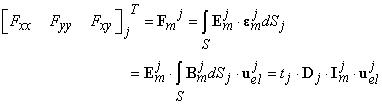(1)

and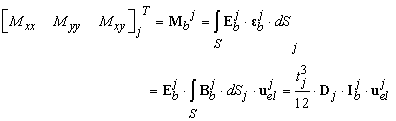(2)

The auxiliary matrices Im and Ib can be calculated only using the numerical approach. Further details about Em, Eb, D, Bm, Bb, uel and t are presented in [10, 11]. The extreme stress values can be expected at the top or at the bottom surface. Generally, it means[8, 10].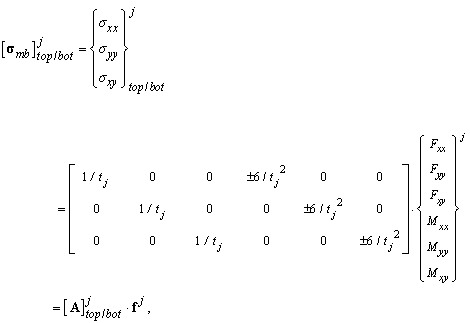(3)

fortop or bottom element surface. Generally, the “top” or “bottom” von Mises stresses may be calculated from relations [5, 6, 10]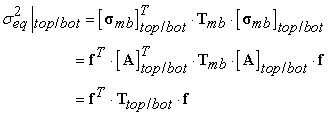(4)

where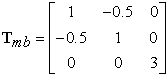(5)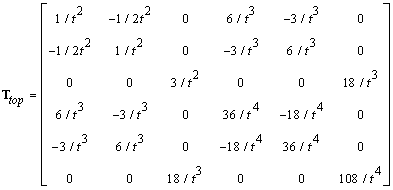(6)

and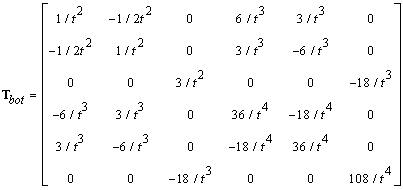(7)

### 3. Proposed Numericalalgorithms

FSD is based on the assumption of independence between axial forces and size of cross-section areas in statically determined truss structures, while this assumption shows good results while optimizing truss structures, it has some drawbacks while using shell elements. Problems can arise when stiffness of structure is changed by altering thicknesses of neighboring optimization groups [5, 9]. These problems lead to need of higher number of iteration steps used to reach solution satisfying convergence conditions. Authors divided the proposed algorithms into two groups; the first three algorithms are based solely on prediction of equivalent stress, while another four are based on prediction of internal forces. For purpose of unity between algorithms, all of them are based on comparing predicted equivalent stress for each allowed thickness with stress limit and the smallest thickness satisfying condition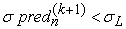is chosen.

3.1. 1st Algorithm - the "Classical" Approach FSD (Regula-Falsi Method)

In engineering mechanics it is used mainly in design of truss structures .The geometric interpretation of the method is shown in Figure 1. Starting from similarity of triangles OAB and OCD we will get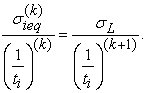(8)

From this equation we obtain well-known iterative relation (9). Mentioned geometric interpretation shows, that new estimation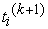is approximated from points [0, 0] and [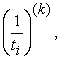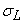]. From the numerical mathematics point of view we are speaking about Regula-Falsi method (method of chords-secants) [8, 11]. FSD iteration relation can be derivedfrom these equations as follows: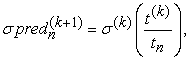(9)

Where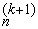is predicted stress for thickness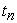in (k+1)th step,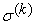is stress in kth step tor thickness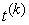,is thickness in kth step,isnth thickness from vector of allowed thicknesses.

It means if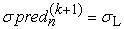then tk+1 is “the best value from tn values” for condition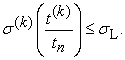3.2. 2nd Algorithm - "Squared" FSD Method

This method originated from the "classical" approach (Alg. No. 1). Previous numerical tests proved instability of algorithm No. 1 and an interesting behavior was observed. The "classical" approach seemed to oscillate and slowly approach the optimum, the goal of this improved so called "squared" FSD method is to smooth the progress of changes of new variable values, thus minimize oscillation and accelerate convergence to the optimum . The predicted equivalent stress is obtained by formula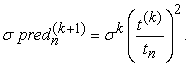(10)

Despite similarity between formula used in this and the one used in the previous algorithm, the addition of the second exponent proved to make significant influence on the results. Numerical tests showed high stability when optimizing models with higher number of optimization groups while preserving reasonable speed.

3.3. 3rd Algorithm - Newton’s Methodforpredictionof Equivalent Stress

This method is very similar to Newton's tangent method [6, 8, 9]. The problem of acquiring derivation of an unknown relation between thickness and equivalent stress of particular optimization group was solved by Newton numerical algorithm. As we lack preceding results (from (k-1)th step) in the first iteration, we have to use another method to acquire the first set of results and the vector of thicknesses used in the second analysis.

Geometric interpretation of the method is shown in Figure 3. Starting from similarity of triangles ABC and BDE we will get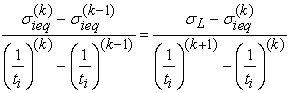(11)

and after modification we obtain following iterative relation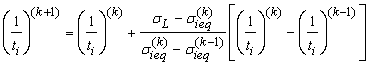(12)

However, as stated before, we based our methods on stress prediction, so we used following iterative relation for prediction of von Mises equivalent stress. Predicted stress in (k+1)th iteration for thickness tn is calculated as follows: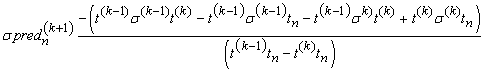(13)

Where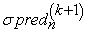is predicted stress for thickness tn in (k+1)th stepis stress in kth step for thickness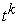,is thickness in kth step,= nth thickness from vector of allowed thicknesses,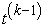isthickness in (k-1)th step,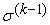is stress in (k-1)th step.

This method proved to be very fast while dealing with relatively small number of variables, although with larger number of variables stability problems can arise preventing successful finding of solution.

3.4. 4th Algorithm - Linear Approximation of Internal Forces and Moments

The fourth proposed algorithm is using linear approximation to predict membrane forces and bending moments for thickness tn assuming linear relation between force quantity and inverse of thickness. Numerical tests proved this method to be reliable, without stability problems and preserving speed while optimizing both small and large number of optimization groups. Predicted membrane forces and bending moments are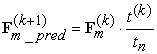(14)

and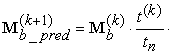(15)

Predicted stresses on the top and bottomcan be expressedas follows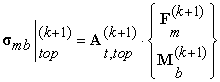(16)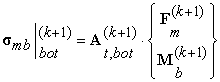(17)

Predicted equivalent stress is calculated using von Mises hypothesis (Eq. 4).

3.5. 5th Algorithm-Newton’smethod for Prediction of Internal Forces and Moments

An approach similar to the third algorithm was implemented for prediction of internal forces and moments.Geometric interpretation of the method is shown in Figure 5. Following relations are based on similarity of triangles ABC and BDE.Predicted membrane forces and bending moments will be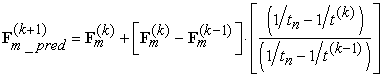(18)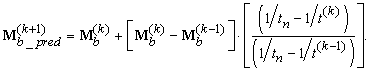(19)

Predicted stresses are calculated using (9), (10) and (4).

3.6. 6th Algorithm-“Squared" Approximation of Internal Forces and Moments

As addition of second exponent in the algorithm No. 2 proved to be significant improvement to "classical" approach of the FSD method, authors also tested similar change to algorithm No. 4 (linear approximation of internal forces) and added square root to relationship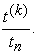Predicted membrane forces and bending moments: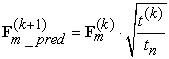(20)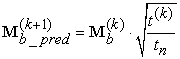(21)

Predicted stresses are calculated using equations (9), (10) and (4).

3.7. 7th Algorithm-"Mixed" Approximation of Internal Forces and Moments

Algorithm No. 7 is a combination of linear approximation of membrane forces from algorithm No. 4 and nonlinear approximation of bending moments from algorithm No. 6.

Predicted membrane forces and bending moments:(22)(23)

Predicted stresses are calculated using (9), (10) and (4).

### 4. Testing Example

Presented seven computational algorithms were tested and compared on cranked beam Figure 1. The 3-node triangular shell elements were used. Number of finite elements was 5462 and number of nodes was 5739.The material model was linear elastic isotropic steel withYoung's modulus of elasticity E=2,1.105 MPa, Poisson's ratioν=0,3, stress limit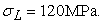Following boundary conditions were used:

- load conditions: pressure p = 1.0 MPa on three quarters of upper flange,

- deformation conditions: fixed left side.

### 5. Results

The results of analyses present means of improving the classical formulation of the FSD method. It has to be noted that the increase of effectiveness is not as significant as authors expected. Summary of iteration steps needed is presented in Table 1 and Figure 9. History of mass convergence of individual algorithms is in Figure 10. The Figures. 11 until 17 present the history of convergence of the equivalent stresses represented each optimizing group.

#### Table 1. Summary of iteration steps numberDownload asPowerPoint Slide

Veiw figureView current table in a new window

### 6. Conclusion

Our paper summarizes study of effectiveness of algorithms based on the FSD principle applied on computational models of thin shell finite elements. Using principles of numerical mathematics and theoretical basics of stress calculation for shells were proposed new computational algorithms which authors tested on various problemsand for different types of finite elements.Numerical tests show relatively similar behavior of algorithms 2, 4, 6 and 7 in terms of stability when dealing with large number of optimization variables, although speed varied between various testing examples.Authors practice showed small increase in effectiveness of some algorithms but significant improvement has not been seen yet.The presented computational procedures were inbuilt into MATLAB’s software module MAT_FSD which cooperates with FE software ADINA.

### Acknowledgement

This work has been supported by VEGA grant No. 1/0234/13.

### References

  Arora, J.S., Huang, M.W., “Discrete structural optimization with commercially available sections: A review”, Structural Earthquake Eng. JSCE, No. 13, 93-110, 1996.In article  Bathe, K.J.,Finite Element Procedures,Prentice Hall, New Persey, 1996.In article  Dekýš, V., Sapietová, A., Kocúr, R. “On the reliability estimation of the conveyer mechanism using the Monte Carlo method”, in Proc. COSIM 2006, Krynica-Zdroj, 2006, 67-74.In article  Haftka, R.T., Gürdal Z., Elements of Structural Optimization, Kluwer Academic Publisher, 1992.In article CrossRef  Jakubovičová, L., Kopas, P., “Contribution to Stress and Residual Strain Analyse of the Welded Specimen”, Transactions of the University of Košice, No. 3, 2012, 57-64.In article  Kwon, Y.W., Bang, H.,The Finite Element Method using MATLAB, CRC Press University of Minnesota, Minnesota, 1996.In article  Leitner, B., Kopas, P., “The vector autoregressive moving average models asa tool for stochastically loaded dynamic systems identification”, Machine Dynamics Research, No. 4, Vol. 34, 32-41, 2010.In article  Sága, M., Dudinský, M. Pecháč, P.,“Optimization of thin shell structures using FSD algorithms”, Communications, Vol. 14, No. 3, 32-38, 2012.In article  Sága, M., Dudinský, M., Pecháč, P., “Numerical study of the new FSD algorithms in the case of the shell FE models”, in Machine Modeling and Simulations, 2012, 5-12.In article  Sága, M., Vaško, M., “Stress Sensitivity Analysis of the Beam and Shell Finite Elements”, Communications, Vol. 11, No. 2, 5-12, 2009.In article  Sága, M., Vaško, M., Kocúr, R., Toth, Ľ., Kohár, R., Application of optimizing algorithms in solid mechanics (in Slovak), VTS ŽU Žilina, Žilina, 2006.In article  Sapietová, A., Dekýš, V., “A design and optimization of the fully automatic shunting mechanism”, in Advances in Mechanisms Design, Mechanisms and Machine Science 8, Vol. 10, 2012,421-427.In article  Žmindák, M., Novák, P., “Particles Interactions in Composites Reinforced by Fibre and Spherical Inclusions”, Communications, Vol. 11, No. 2, 13-18, 2009.In article